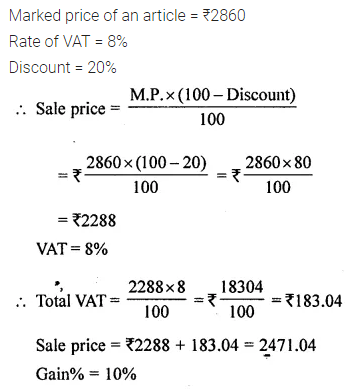# ML Aggarwal Class 8 Solutions for ICSE Maths Chapter 7 Percentage Objective Type Questions

## ML Aggarwal Class 8 Solutions for ICSE Maths Chapter 7 Percentage Objective Type Questions

Mental Maths
Question 1.
Fill in the blanks:
(i) The percentage is the numerator of a fraction with denominator …………..
(ii) 15% of ………….. is 135.
(iii) $$\frac{3}{4}$$ = ………..%
(iv) 50 paise = ………….. % of 8 rupees.
(v) A shopkeeper earns a profit if S.P. is ………….. than C.P.
(vi) Loss or profit % is always calculated on ………….. price.
(vii) The additional expenses on transportation, labour, repairing etc. are called …………..expenses.
(viii)Amount deducted from the marked price of an article is called ……..
(ix) Discount = Marked price – ………….. price.
(x) These days, sales tax is known as …………..
Solution:Question 2.
State whether the following statements are true (T) or false (F):
(i) Discount is always calculated on the marked price.
(ii) Two successive discounts of 20% and 20% are equivalent to a single discount of 40%.
(iii) Overheads are always added to the original cost to get the total cost.
(iv) If S.P. is less than C.P. then shopkeeper suffers a loss.
(v) Sales tax is always calculated on the selling price.
Solution:Multiple Choice Questions
Choose the correct answer from the given four options (3 to 18):
Question 3.
$$3 \frac{1}{8}$$ % of 75 kg is equal toSolution:Question 4.
$$2 \frac{1}{12}$$ is equivalent to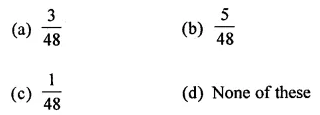Solution:Question 5.
$$1 \frac{11}{16}$$ is equivalent to
(a) 168.75%
(b) 169.75%
(c) 170.75%
(d) 167.75%
Solution:Question 6.
21 : 80 is equivalent to
(a) 26%
(b) 26.50%
(c) 26.75%
(d) 26.25%
Solution:Question 7.
If 5% of a number is 42, then the number is
(a) 800
(b) 820
(c) 840
(d) 860
Solution: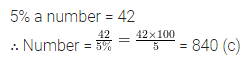Question 8.
What % of 150 is 30?
(a) 50%
(b) 10%
(c) 15%
(d) 20%
Solution:Question 9.
A number increased by 25% gives 155. The number is
(a) 132
(b) 128
(c) 124
(d) 120
Solution:Question 10.
Naman buys a game for ₹60 and sells it for ₹75, his profit % is
(a) 15%
(b) 20%
(c) 25%
(d) 30%
Solution: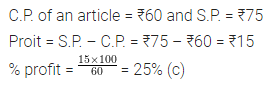Question 11.
Cost price of a watch is ₹680. If Rishabh sells it at a profit of 35%, then S.P. is
(a) ₹918
(b) ₹919
(c) ₹920
(d) ₹921
Solution:Question 12.
On selling an article for ₹ 150, Renu gains ₹30. Her gain percentage is
(a) 15%
(b) 20%
(c) 25%
(d) 30%
Solution:Question 13.
If the cost price of 5 articles is equal to the selling price of 6 articles, then there is
(a) gain
(b) loss
(c) no gain no loss
(d) none of these
Solution: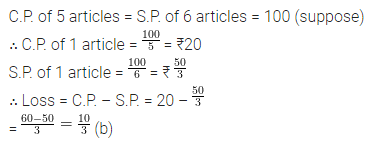Question 14.
Marked price of an article is ₹675. If it is sold at a discount of 20%, then S.P. is
(a) ₹540
(b) ₹525
(c) ₹510
(d) ₹500
Solution:Question 15.
A jacket is marked for ₹2590. If the S.P. of the jacket is ₹2331, then discount % is
(a) 5%
(b) 10%
(c) 15%
(d) 20%
Solution:Question 16.
Marked price of a showpiece is ₹950. S.P. of showpiece after two successive discounts of 10% and 10% is
(a) ₹ 769.50
(b) ₹ 760
(c) ₹ 855
(d) None of these.
Solution:Question 17.
S.P. of a video game is ₹749 including 7% VAT. The original price of a video game is
(a) ₹801.43
(b) ₹742
(c) ₹700
(d) None of these
Solution:Question 18.
List price of an article is ₹1050. If 6% sales tax is charged, then bill amount is
(a) ₹1056
(b) ₹1113
(c) ₹1131
(d) ₹1311
Solution:Value Based Questions
Question 1.
Out of 500 students in a school, 60% of students read Hindi Newspaper, 30% of students read English Newspaper and remaining students do not read any newspaper. Find
(i) What % of students do not read any newspaper?
(ii) Number of students who read Hindi newspaper.
(iii) Number of students who read English newspaper.
Is reading the newspaper a good habit? What are the advantages of the reading newspaper?
Solution:Question 2.
In an exam at least 35% marks are required to pass the exam. Ramesh uses unfair means and tries to pass the exam but fails by 15 marks. If he scored 160 marks, find the maximum marks.
Is using unfair means in the exam is good? Why should we not use unfair means?
Solution:Question 3.
A shopkeeper was involved in tax evasion. To save sales tax he never gives receipts to his customers. He sold an article for ₹550 including 10% VAT. Find the amount of VAT not paid by him to the Government. Is tax evasion correct? Why should we pay tax? What measures should we adopt to stop tax evasions?
Solution:Higher Order Thinking Skills (Hots)
Question 1.
Marked price of an article is ₹2860 and rate of VAT is 8%. A shopkeeper allows a discount of 20% and still makes a profit of 10%. If he spent 4% as overheads, then find the original cost price of the article and final selling price including VAT.
Solution: